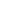XTransform ClassRepresents a world space transform. When first created the object defaults to the identity - no transformation. A world space transform is not same an object transform. You are changing the coordinate system - not the objects you're inserting. ```System.Object    WebSupergoo.ABCpdf12.XTransform Implements: IDisposable, IEquatable, IComparable```

 Method Description XTransform XTransform Constructor. Invert Invert the transform. Equals Determines if two transforms are effectively the same. Magnify Scale about a locked anchor point. PreMultiply Pre-multiplies this transformation matrix by the supplied transform. PostMultiply Post-multiplies this transformation matrix by the supplied transform. Reset Reset to the identity. Rotate Rotate about a locked anchor point (angle in degrees). Skew Skew horizontally and vertically about a locked anchor point. SetTransform Set the transform. ToString Returns a string representation of the object. TransformPoint Applies this transform to a specified point. TransformPoints Applies this transform to a specified array of points. Translate Translate horizontally and vertically. GetHashCode A hash code for the XTransform.

 Property Description String The transform as a string. AngleUnit The angle unit, degrees or radians. Elements The transform as an array of floating-point values. Matrix The transform as a System.Drawing.Drawing2D Matrix. MediaMatrix The transform as a System.Windows.Media Matrix. OffsetX The x translation. OffsetY The y translation.